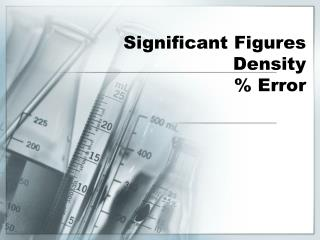DownloadDownload PresentationSignificant Figures Density % Error

# Significant Figures Density % Error

Download Presentation## Significant Figures Density % Error

- - - - - - - - - - - - - - - - - - - - - - - - - - - E N D - - - - - - - - - - - - - - - - - - - - - - - - - - -
##### Presentation Transcript

1. Significant FiguresDensity% Error

2. Significant Figures • The number of digits reported in a measurement. • All the known digits plus one estimated value. • The number of significant figures possible depends upon the piece of equipment used to take the measurement.

3. Significant Figures

4. Rules for Significant Figures • Non-zero numbers are always significant. • Zeros between non-zeros are always significant. • All final zeros to the rightof the decimal place are significant. • Zeros that act as placeholders are NOT significant. • Counting numbers and defined constants have an infinite number of significant figures.

5. Determine the number of sig figs in the following numbers. • Red numbers=significantBlack numbers=not significant Practicing Significant Figures • 0.02 • 70001 • 5600 • 4.100 • 3.1416 (π) • 2.80 x 105 • 0.02 • 70001 • 5600 • 4.100 • 3.1416 (π) • 2.80 x 105 • 1 • 5 • 2 • 4 • Infinite • 3

6. Rules for Rounding If the digit to the immediate right of the last sig fig is 5-9, round up. If not, leave as is.

7. Significant Figures and Calculators When using a calculator, you should do the calculation using the digits allowed by the calculator and round off only at the end of the problem. Do not round off in the middle of the problem!

8. Sig Figs and Addition/Subtraction + - + - + - + - + - + - + - + When you add or subtract, you answer must have the same number of digits to the right of the decimal point as the original value with the fewest digits to the right of the decimal place. + - + - + - + - + - + - + - +

9. Sig Figs and Multiplication/Division When you multiply or divide, your answer must have the same number of significant figures as the original value with the least significant figures.

10. Practicing Significant Figures 3.33 m2 25 m 53 mL 26.6 g 6.7 cm3

11. Density

12. Which weighs more, a pound of bricks or a pound of feathers?

13. Density • When something feels heavy, you are noting its density. • Density—the mass of an object per unit volume

14. Density m v D= • The unit for density should always be some unit for mass over some unit for volume. • Usually it is g/mL, g/L or kg/m3

15. Practice Problem #1 • What is the density of an object that has a mass of 22.5g and a volume of 30.4 mL?

16. Practice Problem #2 • If a cube has sides that are 8.2 mL each and a total mass of 46.1g, what is its density?

17. Practice Problem #3 • What is the volume of isopropyl alcohol if its mass is 45.22g and it has a density of 0.899 g/mL?

18. Percent Error A way to evaluate the accuracy of data. Percent Error=Ratio of the error to the accepted value │Accepted value – Measured value│ Accepted value X 100%

19. 125.0 mL – 123.4 mL 1.6 mL ________________________________________ _______________ 125.0 mL 125.0 mL = = 1.3% X 100% Percent Error If your measurement of a liquid is 123.4 mL but the actual amount is 125.0 mL, what is the percent error of the measurement?